## Solution to 1986 Problem 76

 The initial kinetic energy of the ball is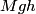$Mgh$. The final kinetic energy is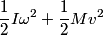\begin{align*}\frac{1}{2} I \omega^2 + \frac{1}{2}M v^2\end{align*}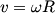$v = \omega R$ and for a hoop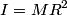$I = M R^2$, so the final kinetic energy is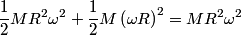\begin{align*}\frac{1}{2} M R^2 \omega^2 + \frac{1}{2}M \left(\omega R\right)^2 = M R^2 \omega^2\end{align*}So,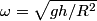\begin{align*}\omega = \sqrt{g h /R^2}\end{align*}The angular momentum about the center of mass is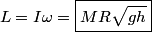\begin{align*}L = I \omega = \boxed{M R \sqrt{g h}}\end{align*}Therefore, answer (A) is correct.